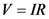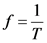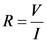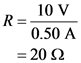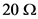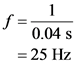# The figure shows voltage and current graphs for a resistor.(figure 1)

The figure shows voltage and
current graphs for a resistor.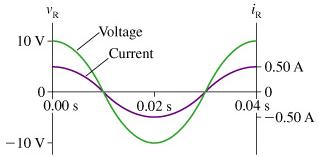Part A –
What is the value of the resistance R ?
Express your answer using two significant figures.
R = _______Ω
Part B –
What is the emf frequency f ?
Express your answer using two significant figures.
f = __________Hz

General guidance

Concepts and reason
The concepts required to solve this problem are the Ohm’s law and frequency.
Initially, use the Ohm’s law. Rearrange the expression for the resistance. Later, using the time from the given figure, calculate the emf frequency.

Fundamentals

The expression of the ohm’s law is,

Here, V is the voltage, I is the current, and R is the resistance.
The expression of the frequency is,

Here, f is the frequency and T is the time.

Step-by-step

Step 1 of 2

(A)
Refer the figure given in the question.
The expression of the ohm’s law is,

Rearrange the expression of the resistance.

Substitute 10 V for V and 0.50 A for I.

Part A
The resistance is .

The Ohm’s law states that the potential difference across a conductor is directly proportional to the current flowing through it. The resistance is the proportionality constant.

Divide the voltage by the current to find the resistance.

Step 2 of 2

(B)
Refer the figure given in the question.
The expression of the frequency is,

Substitute 0.04 s for T in the above equation.

Part B
The emf frequency is 25 Hz.

The time and the frequency are related reciprocally. The number of oscillations per unit time is known as frequency. The units are hertz’s.

Part A
The resistance is .

Part B
The emf frequency is 25 Hz.